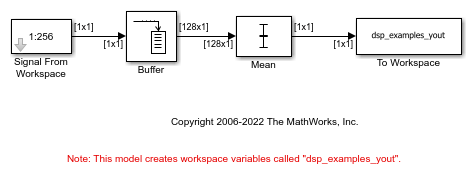# Compute Mean Using Sliding Window

Compute mean of a signal using the Mean block. The Buffer block in this example creates a sliding window to compute the mean.

Open and run the model.

The Buffer block has Output buffer size (per channel) parameter set to 128 and the Buffer overlap parameter set to 127. Using these parameters, the Buffer block creates a sliding window of length 128. The window advances by one sample each time step. The Mean block computes the mean of the buffered data across each column.

The model stores the mean data computed at every time step in the output workspace variable `dsp_examples_yout`.